Function Repository Resource:

# NFunction

Allow a function to perform pure numeric computation by silently ignoring non-numeric values

Contributed by: Ting Sun
 ResourceFunction["NFunction"][fun] makes a special operator form of fun that performs pure numeric computation by ignoring non-numeric values.

## Details

ResourceFunction["NFunction"][fun] mimics the behavior of fun, but performs computations only for numeric values; all non-numeric values are ignored.
When applied to purely numeric data arrays, ResourceFunction["NFunction"][fun] and fun give identical results.
When applied to an empty data array, ResourceFunction["NFunction"][fun] gives Indeterminate.
Functions processed by ResourceFunction["NFunction"] behave similarly to numpy nan-family functions.

## Examples

### Basic Examples (2)

Mean processed by NFunction will ignore non-numerical values when applied to a list:

 In:=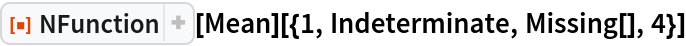Out=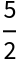For arrays with rank ≥ 2, NFunction is applied columnwise:

 In:=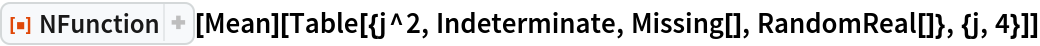Out=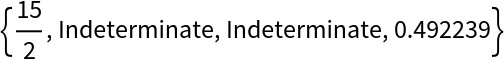### Scope (6)

Custom functions can be processed by NFunction:

 In:=Out=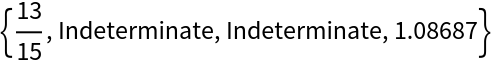Functions processed by NFunction can work with Dataset:

 In:=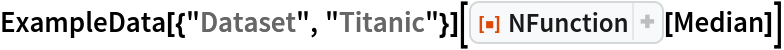Out=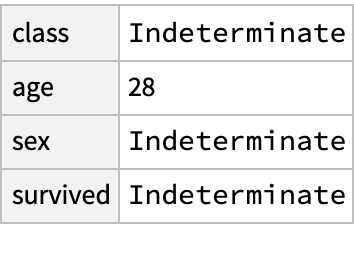Functions processed by NFunction can work with Quantity:

 In:=Out=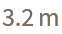Functions processed by NFunction can work with Around:

 In:=Out=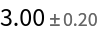Functions that produce results similar to List can also be processed by NFunction:

 In:=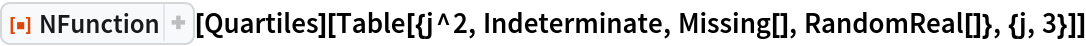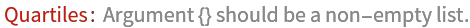Out=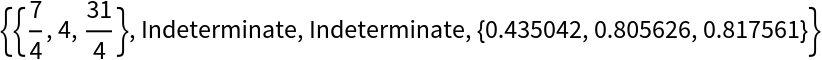Non-numeric results are given as Indeterminate:

 In:=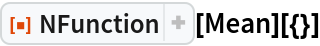Out=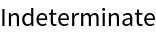### Properties and Relations (1)

Functions processed by NFunction give the same results as those without processing when applied to numeric data arrays:

 In:=Out=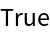### Possible Issues (1)

Some functions require a minimum number of valid values:

 In:=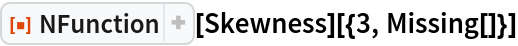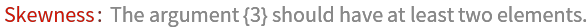Out=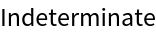Ting Sun

## Version History

• 4.0.0 – 07 September 2021

## Author Notes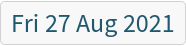: add support for functions that produce List-like results.